$$\renewcommand{\tr}{{#1}^{\mkern-1.5mu\mathsf{T}}}$$ $$\renewcommand{\ve}{\mathbf{#1}}$$ $$\renewcommand{\sv}{\boldsymbol{#1}}$$ $$\renewcommand{\pop}{\mathcal{#1}}$$ $$\renewcommand{\samp}{\mathcal{#1}}$$ $$\renewcommand{\imply}{\Longrightarrow}$$ $$\renewcommand{\given}{~\vert~}$$ $$\renewcommand{\suchthat}{~:~}$$ $$\renewcommand{\widebar}{\overline{#1}}$$ $$\renewcommand{\wig}{\tilde{#1}}$$ $$\renewcommand{\bigwig}{\widetilde{#1}}$$ $$\renewcommand{\field}{\mathbb{#1}}$$ $$\renewcommand{\Reals}{\field{R}}$$ $$\renewcommand{\abs}{\left\lvert ~{#1} ~\right\rvert}$$ $$\renewcommand{\size}{\left\lvert {#1} \right\rvert}$$ $$\renewcommand{\tr}{{#1}^{\mkern-1.5mu\mathsf{T}}}$$ $$\renewcommand{\norm}{\left|\left|{#1}\right|\right|}$$ $$\renewcommand{\intersect}{\cap}$$ $$\renewcommand{\union}{\cup}$$

Note that eikosograms are grid objects and so may be manipulated as any other (e.g. arranging several eikosograms in the same display using grid.arrange() from the gridExtra package).

library(eikosograms)
library(gridExtra)

# A picture of probability

The word eikosogram is constructed by joining two ancient Greek words:

eikos: like truth, likely, probable, reasonable, probability, likelihood
gramma: that which is drawn, picture, piece of writing

As the name suggests, an eikosogram is a picture of probability. It visually partitions a unit square into rectangular regions whose areas give the numerical values of various probabilities. The construction is such that each rectangular region is identified with the value of one or more categorical variates.

For example, consider the probability that an application for admission to university is accepted. Different universities and programs of study can have different admission rates. For example, in 2017 there were about 15,200 applications to the Faculty of Mathematics at the University of Waterloo for only 1200 first year places, suggesting the probability that an application is accepted is about 0.08. Suppose this to be the case, then the eikosogram representing the application process is given by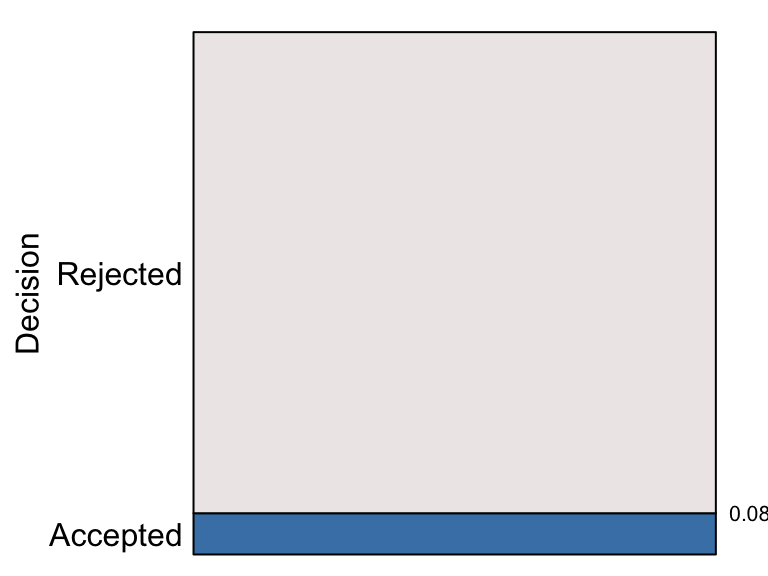Application to Waterloo’s Faculty of Mathematics (2017)

Only two decisions are possible – an application is either accepted (and an offer of admission made) or it is rejected, as identified by the abels on the left side of eikosogram. On the right side of the eikosogram a single number appears, 0.08, marking the vertical height of the bottom (blue) rectangle.

An eikosogram always has unit area (whatever its aspect ratio) and represents the totality of its probability with each side of the eikosogram extending from 0 to 1. The bottom (blue) rectangle is associated with the event that an application is accepted and its area the probability of acceptance. This probability is determined by simply calculating this area from the eikosogram as $\begin{array}{rcl} Pr(Decision = Accepted) & = & width \times height \\ && \\ & = & 1 \times 0.08 \\ && \\ & = & 0.08 \end{array}$ Since Decision is a binary variate, the top (grey) rectangle is associated with the application being rejected. The area of this rectangle is the probability $\begin{array}{rcl} Pr(Decision = Rejected) & = & width \times height \\ && \\ & = & 1 \times (1 - 0.08) \\ && \\ & = & 0.92 . \end{array}$ These two areas give the probability distribution of the binary random variate Decision whose value must be one of $$\{Accepted, ~Rejected \}$$. The eikosogram gives a visual representation of this probability distribution; the relative areas visually convey the magnitude of the probabilities. Moreover, since the width of both rectangles is the same (viz. 1) these probabilities are also given more simply (and visually more accurately) by the heights of the two rectangles.

A well known data set in R is UCBAdmissions which recorde the number of applications and admissions to several large graduate at the University of California (Berkeley) in 1973. This is a table of counts cross classified by three different factors:

##  'table' num [1:2, 1:2, 1:6] 512 313 89 19 353 207 17 8 120 205 ...
##  - attr(*, "dimnames")=List of 3
##   ..$Admit : chr [1:2] "Admitted" "Rejected" ## ..$ Gender: chr [1:2] "Male" "Female"
##   ..\$ Dept  : chr [1:6] "A" "B" "C" "D" ...

The eikos() function is used to produce the eikosogram showing the probability of admission,

eikos("Admit", data = UCBAdmissions)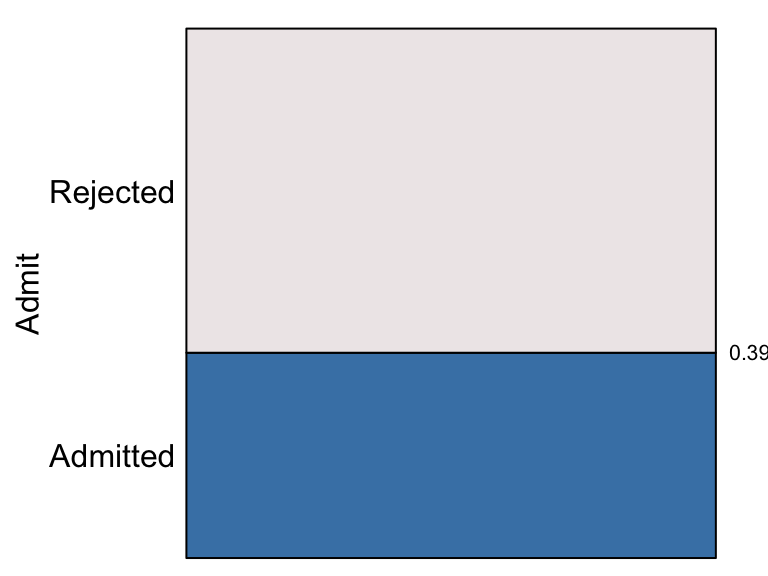The proportion of applications admitted to these graduate programs at Berkeley in 1973 was 0.39. The probability of an application being admitted is represented by the area (or height) of the bottom (blue) rectangle and the probability of rejection by the area (or height) of the top (grey) rectangle which is 1 - 0.39 = 0.61.

The first argument to eikos() is the y or “response” variate and determines the positions on the vertical axis. There will be as many horizontal rectangles stacked up the vertical axis as there are distinct values for y. For example, the probability that any application is submitted to each of the six graduate departments is

eikos("Dept", data = UCBAdmissions)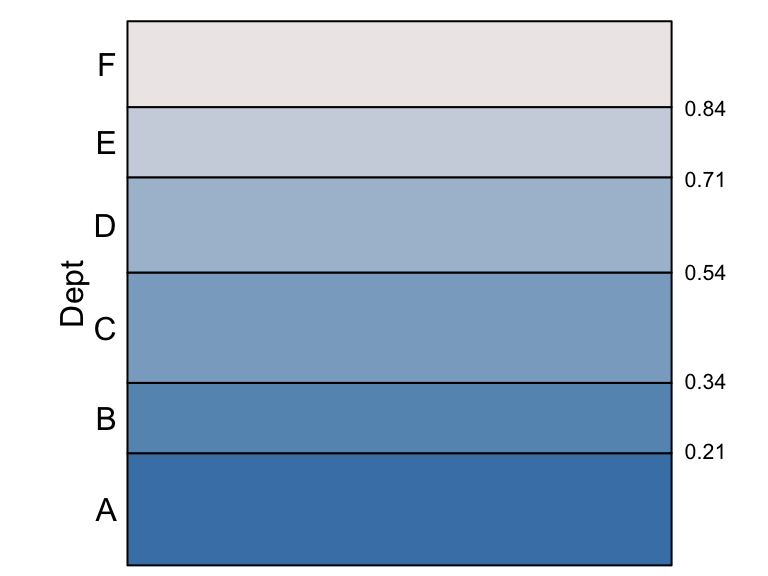Again, areas correspond to probabilities and can be determined in this case from each rectangle’s height (since widths are all 1). The probability that a randomly selected application was submitted to Department A is 0.21, to B is 0.13 (= 0.34 - 0.21), to C is 0.20 (= 0.54 - 0.34), to D is 0.17 (= 0.71 - 0.54), to E is 0.13 (= 0.84 - 0.71), and finally to F is 0.16 (= 1 - 0.84).

# Conditional and marginal probabilites

The eikosogram reinforces the basic probability rule that when the values of the variate are mutually exclusive and exhaust the set of possibilities, the probabilities must sum to one: $Pr(Dept = A) + Pr(Dept = B) + \cdots + Pr(Dept = F) = 1$ Note that this was because not all Berkeley departments are being considered here; these probabilities are therefore conditional on the fact that the department is one of the six largest.

In a very direct sense, the unit square of the eikosogram frames the probabilities expressed within it and these are always conditional on whatever background information determines the unit square.

For example, in addition to considering only the six largest departments, we could also restrict consideration by sex. The number of applications from each gender is

eikos("Gender", data = UCBAdmissions)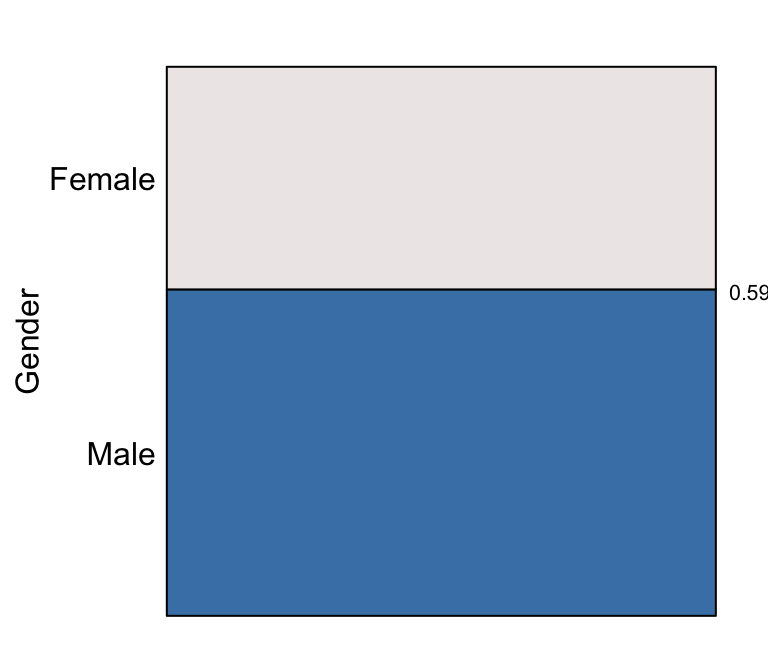showing that there are unequal numbers of applications from each sex with $$Pr(Gender = Male) = 0.59$$ and $$Pr(Gender = Female) = 0.41$$.

The eikosogram conditional only on “Male” applicants is

eikos("Dept", data = UCBAdmissions[,"Male",],
main = "Applications from males")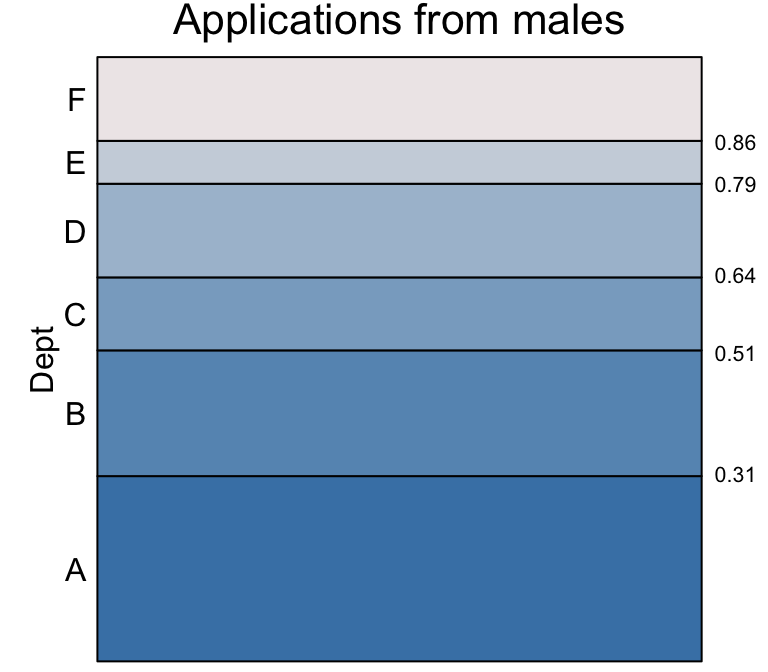Probabilities can be determined as before but we can see immediately from the eikosogram that departments A and B are the most popular departments for male applicants, more so than for all applications combined (in the previous eikosogram).

Conditioning on the “Female” applications

eikos("Dept", data = UCBAdmissions[,"Female",],
main = "Applications from females")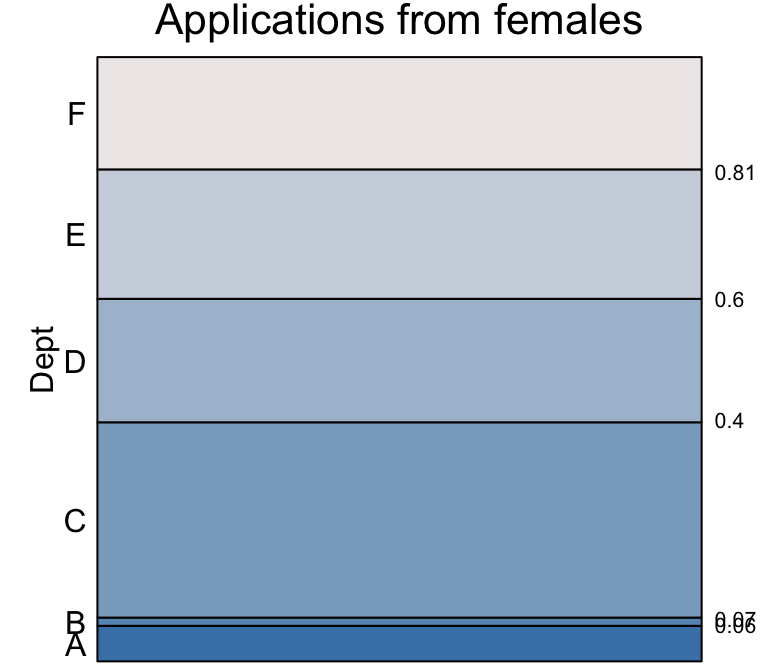we see a rather different distribution of probabilities. Departments A and B are much less popular with female applicants than with male (e.g. only 1 in 100 applications from females are to B compared to 1 in 5 for males). Instead departments C and E are much more likely to receive an application if it is from a female than if it is from a male.

Knowing the marginal probabilities that an application is from a female or a male, we might put these two eikosograms together in a single eikosogram.

eikos(y = "Dept", x = "Gender",  data = UCBAdmissions,
xprobs_size = 8, yprobs_size = 8)